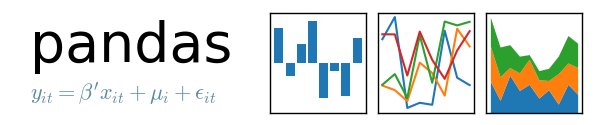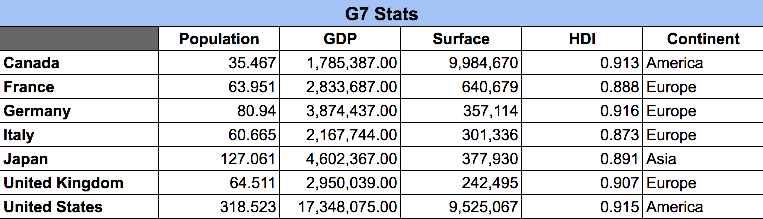# Intro to Pandas DataFrames

Last updated: November 28th, 2019# Intro to Pandas DataFrames¶

Probably the most important data structure of pandas is the DataFrame. It's a tabular structure tightly integrated with Series.

A DataFrame is a tabular structure with the following properties:

• It's composed by a ordered series of rows and a ordered series of columns.
• It also uses an index to reference individual rows.
• Each column could have a different NumPy-related type.
• It could be seen as a collection of multiple of Series, all sharing the same index.
• Can be "sliced" horizontally (per row) or vertically (per column).## Hands on!¶

In :
import numpy as np
import pandas as pd## DataFrames creation¶

The DataFrame constructor accepts the following parameters:

• data: (required) has all the data we want to store on the DataFrame and could be a Series dictionary, a sequences dictionary, a bidimensional ndarray, a Series or another DataFrame.
• index: (optional), has all the labels we want to assign to the rows of our DatFrame and could be a Python sequence or an unidimensional ndarray. Default value: np.arange(0, len(rows)).
• columns: (optional), has all the labels we want to assign to the columns of our DatFrame and could be a Python sequence or an unidimensional ndarray. Default value: np.arange(0, len(columns)).
• dtype: (optional) any NumPy data type to be assigned on columns.
In :
# Using a dictionary of sequences
dataframe = pd.DataFrame({'var1': [1, 2, 3],
'var2': ['one', 'two', 'three'],
'var3': [1.0, 2.0, 3.0]})

dataframe

Out:
var1 var2 var3
0 1 one 1.0
1 2 two 2.0
2 3 three 3.0
In :
# Using a dictionary of Series
dataframe = pd.DataFrame({'var1': pd.Series([1, 2, 3], dtype=np.float64),
'var2': pd.Series(['a', 'b'])})

dataframe

Out:
var1 var2
0 1.0 a
1 2.0 b
2 3.0 NaN
In :
# Using a ndarray with indexes to rows and columns
dataframe = pd.DataFrame(np.arange(16).reshape(4, 4),
index=['r1', 'r2', 'r3', 'r4'],
columns=['c1', 'c2', 'c3', 'c4'])
dataframe

Out:
c1 c2 c3 c4
r1 0 1 2 3
r2 4 5 6 7
r3 8 9 10 11
r4 12 13 14 15
In :
# Using a ndarray with indexes to rows and columns, with fixed type
dataframe = pd.DataFrame(np.arange(16).reshape(4,4), dtype=np.int32)

dataframe

Out:
0 1 2 3
0 0 1 2 3
1 4 5 6 7
2 8 9 10 11
3 12 13 14 15
In :
dataframe.dtypes

Out:
0    int32
1    int32
2    int32
3    int32
dtype: object## DataFrame elements¶

DataFrames expose some useful attributes:

In :
dataframe = pd.DataFrame({'var1': pd.Series([1, 2, 3], dtype=np.float64),
'var2': pd.Series(['a', 'b'])})

dataframe

Out:
var1 var2
0 1.0 a
1 2.0 b
2 3.0 NaN
In :
# Show first rows of our DataFrame

Out:
var1 var2
0 1.0 a
1 2.0 b
2 3.0 NaN
In :
# Type of our DataFrame columns
dataframe.dtypes

Out:
var1    float64
var2     object
dtype: object
In :
dataframe

Out:
var1 var2
0 1.0 a
1 2.0 b
2 3.0 NaN
In :
# Values of a DataFrame
dataframe.values

Out:
array([[1.0, 'a'],
[2.0, 'b'],
[3.0, nan]], dtype=object)
In :
dataframe.info()

<class 'pandas.core.frame.DataFrame'>
RangeIndex: 3 entries, 0 to 2
Data columns (total 2 columns):
var1    3 non-null float64
var2    2 non-null object
dtypes: float64(1), object(1)
memory usage: 176.0+ bytes

In :
type(dataframe.values)

Out:
numpy.ndarray
In :
# Using a ndarray with indexes to rows and columns
dataframe = pd.DataFrame(np.arange(16).reshape(4, 4),
index=['r1', 'r2', 'r3', 'r4'],
columns=['c1', 'c2', 'c3', 'c4'])
dataframe

Out:
c1 c2 c3 c4
r1 0 1 2 3
r2 4 5 6 7
r3 8 9 10 11
r4 12 13 14 15
In :
# Index of a DataFrame
dataframe.index

Out:
Index(['r1', 'r2', 'r3', 'r4'], dtype='object')
In :
# Columns of a DataFrame
dataframe.columns

Out:
Index(['c1', 'c2', 'c3', 'c4'], dtype='object')
In :
# Dimension of the DataFrame
dataframe.ndim

Out:
2
In :
# Shape of the DataFrame
dataframe.shape

Out:
(4, 4)
In :
# Number of DataFrame elements
dataframe.size

Out:
16

Indexes are immutable, so we can't change individual values independently. However, we can change a complete index with a new index.

In :
dataframe = pd.DataFrame({'var1': pd.Series([1, 2, 3], dtype=np.float64),
'var2': pd.Series(['a', 'b'])})

dataframe

Out:
var1 var2
0 1.0 a
1 2.0 b
2 3.0 NaN
In :
# Modifying a row index will give us an error
dataframe.index = 4

---------------------------------------------------------------------------
TypeError                                 Traceback (most recent call last)
<ipython-input-21-96113c2111c7> in <module>
1 # Modifying a row index will give us an error
----> 2 dataframe.index = 4

/usr/local/lib/python3.7/site-packages/pandas/core/indexes/base.py in __setitem__(self, key, value)
4258
4259     def __setitem__(self, key, value):
-> 4260         raise TypeError("Index does not support mutable operations")
4261
4262     def __getitem__(self, key):

TypeError: Index does not support mutable operations
In :
# Modifying a column index will give us an error
dataframe.columns = 4

---------------------------------------------------------------------------
TypeError                                 Traceback (most recent call last)
<ipython-input-22-e244ac47fdd7> in <module>
1 # Modifying a column index will give us an error
----> 2 dataframe.columns = 4

/usr/local/lib/python3.7/site-packages/pandas/core/indexes/base.py in __setitem__(self, key, value)
4258
4259     def __setitem__(self, key, value):
-> 4260         raise TypeError("Index does not support mutable operations")
4261
4262     def __getitem__(self, key):

TypeError: Index does not support mutable operations
In :
# This will work
dataframe.index = ['r1', 'r2', 'r3']
dataframe

Out:
var1 var2
r1 1.0 a
r2 2.0 b
r3 3.0 NaN
In :
# This will work
dataframe.columns = ['c1', 'c2']
dataframe

Out:
c1 c2
r1 1.0 a
r2 2.0 b
r3 3.0 NaN## The Group of Seven¶

We'll keep our analysis of "G7 countries" and looking now at DataFrames. As said, a DataFrame looks a lot like a table (as the one you can appreciate here):Creating DataFrames manually can be tedious. 99% of the time you'll be pulling the data from a Database, a csv file or the web. But still, you can create a DataFrame by specifying the columns and values:

In :
df = pd.DataFrame({
'Population': [35.467, 63.951, 80.94 , 60.665, 127.061, 64.511, 318.523],
'GDP': [
1785387,
2833687,
3874437,
2167744,
4602367,
2950039,
17348075
],
'Surface Area': [
9984670,
640679,
357114,
301336,
377930,
242495,
9525067
],
'HDI': [
0.913,
0.888,
0.916,
0.873,
0.891,
0.907,
0.915
],
'Continent': [
'America',
'Europe',
'Europe',
'Europe',
'Asia',
'Europe',
'America'
]
}, columns=['Population', 'GDP', 'Surface Area', 'HDI', 'Continent'])


(The columns attribute is optional. I'm using it to keep the same order as in the picture above)

In :
df.head()

Out:
Population GDP Surface Area HDI Continent
0 35.467 1785387 9984670 0.913 America
1 63.951 2833687 640679 0.888 Europe
2 80.940 3874437 357114 0.916 Europe
3 60.665 2167744 301336 0.873 Europe
4 127.061 4602367 377930 0.891 Asia
In :
df.dtypes

Out:
Population      float64
GDP               int64
Surface Area      int64
HDI             float64
Continent        object
dtype: object
In :
type(df.values)

Out:
numpy.ndarray
In :
df.ndim

Out:
2
In :
df.shape

Out:
(7, 5)
In :
df.size

Out:
35
In :
df.info()

<class 'pandas.core.frame.DataFrame'>
RangeIndex: 7 entries, 0 to 6
Data columns (total 5 columns):
Population      7 non-null float64
GDP             7 non-null int64
Surface Area    7 non-null int64
HDI             7 non-null float64
Continent       7 non-null object
dtypes: float64(2), int64(2), object(1)
memory usage: 408.0+ bytes

In :
df.describe()

Out:
Population GDP Surface Area HDI
count 7.000000 7.000000e+00 7.000000e+00 7.000000
mean 107.302571 5.080248e+06 3.061327e+06 0.900429
std 97.249970 5.494020e+06 4.576187e+06 0.016592
min 35.467000 1.785387e+06 2.424950e+05 0.873000
25% 62.308000 2.500716e+06 3.292250e+05 0.889500
50% 64.511000 2.950039e+06 3.779300e+05 0.907000
75% 104.000500 4.238402e+06 5.082873e+06 0.914000
max 318.523000 1.734808e+07 9.984670e+06 0.916000
In :
df.get_dtype_counts()

/usr/local/lib/python3.7/site-packages/ipykernel_launcher.py:1: FutureWarning: get_dtype_counts has been deprecated and will be removed in a future version. For DataFrames use .dtypes.value_counts()
"""Entry point for launching an IPython kernel.

Out:
float64    2
int64      2
object     1
dtype: int64
In :
df.columns

Out:
Index(['Population', 'GDP', 'Surface Area', 'HDI', 'Continent'], dtype='object')
In :
df.index

Out:
RangeIndex(start=0, stop=7, step=1)`